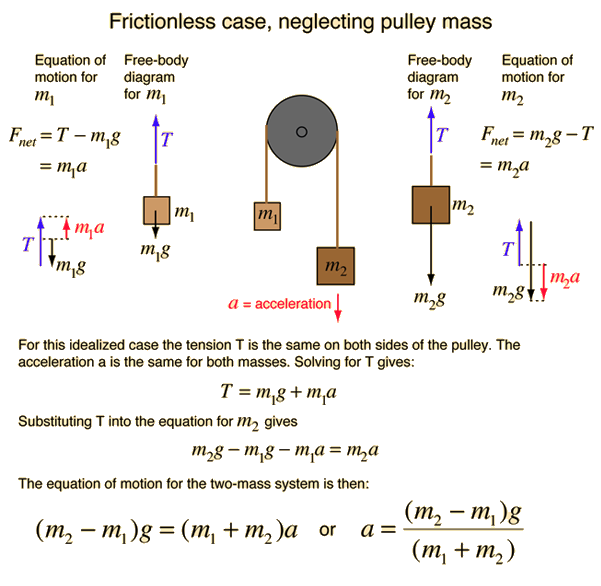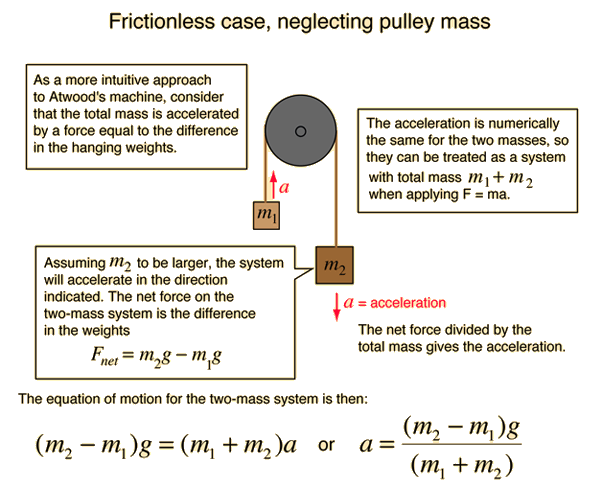# Atwood's Machine### Calculation

Index

Newton's laws

Standard mechanics problems

 HyperPhysics***** Mechanics R Nave
Go Back

# Atwood's Machine### Calculation

Index

Newton's laws

Standard mechanics problems

 HyperPhysics***** Mechanics R Nave
Go Back

# Atwood's Machine

Frictionless case, neglecting pulley mass.

Application of Newton's second law to masses suspended over a pulley: Atwood's machineFor hanging masses: m1 =kg m2 =kg the weights are m1g =N m2g =N The acceleration is a = m/s² and the tension is T = N

Change any of the mass or weight values and the resulting acceleration and tension values will be calculated.

Index

Newton's laws

Standard mechanics problems

 HyperPhysics***** Mechanics R Nave
Go Back